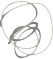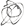Noppanit

11 Nov 2016

# Game of life for dummies

## Game of life

It’s a game invented by the British mathematician John Horton Conway and the game goes like this.


Given a board with m by n cells, each cell has an initial state live (1) or dead (0). Each cell interacts with its eight neighbors (horizontal, vertical, diagonal) using the following four rules (taken from the above Wikipedia article):

Any live cell with fewer than two live neighbors dies, as if caused by under-population.
Any live cell with two or three live neighbors lives on to the next generation.
Any live cell with more than three live neighbors dies, as if by over-population..
Any dead cell with exactly three live neighbors becomes a live cell, as if by reproduction.



Most people know this game because you might be asked during any technical interview to design Game of Life on a whiteboard. But for me, when I first learn how to program I was asked to code Game of Life and it was actually kinda fun. Especially, if you do it in TDD.

## Why this post then?

I know there’s a lot of posts out there solving Game of Life in any possible known computer languages, but I feel like all of them are too hard to understand or use advanced library like numpy to solve the problem which makes it really hard to understand for me. Also, if I would like to understand how to solve the problem and if I can’t explain this simply that means I don’t understand at all.

## Algorithm

The simplest algorithm goes like this.

1. Count all the live cells around 8x8 matrix of a cell.
2. Determine if the next state, the cell lives or dies based on the rules.

So, if you can count all the live cells around a cell, you’re pretty much there.

## Naive approach

class Solution(object):
def gameOfLife(self, board):
"""
:type board: List[List[int]]
:rtype: void Do not return anything, modify board in-place instead.
"""
m = len(board)
n = len(board) if m else 0

temp_board = [ * n for i in range(m)]

def count_cell(i, j):
"""
:type i: Column row
:type j: Column column
Count all the cells around the cell[i][j]
"""

list_of_tuples = [(i-1, j-1), (i-1, j), (i-1, j+1),
(i, j-1), (i, j), (i, j+1),
(i+1, j-1), (i+1, j), (i+1, j+1)]

count = 0
for l in list_of_tuples:
x, y = l
if x >= 0 and y >= 0 and x < m and y < n:
if x is not i or y is not j:
if board[x][y] == 1:
count += 1

return count

for i in range(m):
for j in range(n):
count = count_cell(i, j)
temp_board[i][j] = count

for i in range(m):
for j in range(n):
cell = board[i][j]
count = temp_board[i][j]
if cell == 1:
if count < 2:
board[i][j] = 0
elif count is 3 or count is 2:
board[i][j] = 1
elif count > 3:
board[i][j] = 0
else:
if count == 3:
board[i][j] = 1

board = [[0,0,0,0,0],
[0,0,1,0,0],
[0,0,1,0,0],
[0,0,1,0,0],
[0,0,0,0,0]]

Solution().gameOfLife(board)
print(board)



The naive approach is really simple and stupid. There’s a method to count all the cells and put that to a temp board so it can be used to determined the next cycle of the game.

The complexity would be O(m * n) but the space would be O(m * n) as well

## First refactor

The first refactoring would be to just loop through the surrounding cell with the check for boundaries.


class Solution(object):
def gameOfLife(self, board):
"""
:type board: List[List[int]]
:rtype: void Do not return anything, modify board in-place instead.
"""
m = len(board)
n = len(board) if m else 0

temp_board = [ * n for i in range(m)]

def count_cell(i, j):
"""
:type i: Column row
:type j: Column column
Count all the cells around the cell[i][j]
"""
lives = 0

for I in range(max(i-1, 0), min(i+2, m)):
for J in range(max(j-1, 0), min(j+2, n)):
if I is not i or J is not j:
lives += board[I][J]
return lives

for i in range(m):
for j in range(n):
count = count_cell(i, j)
temp_board[i][j] = count

for i in range(m):
for j in range(n):
cell = board[i][j]
count = temp_board[i][j]
if cell == 1:
if count < 2:
board[i][j] = 0
elif count is 3 or count is 2:
board[i][j] = 1
elif count > 3:
board[i][j] = 0
else:
if count == 3:
board[i][j] = 1

board = [[0,0,0,0,0],
[0,0,1,0,0],
[0,0,1,0,0],
[0,0,1,0,0],
[0,0,0,0,0]]

Solution().gameOfLife(board)
print(board)



It’s a bit better don’t you think?

## Second refactor

Now the first two approaches, we have to have a temp board to calculate the next state. Let’s see if we can do better than that by manipulating the board in-place instead of having a temp board.

Someone on the leetcode discussion suggested bit manipulation to store the state of the cell which I think it’s a brilliant idea. However, I feel like there’s not much explanation so I’m going to try to explain it here.

The problem with the second approach is that we have to have a temp board to store the number of surrounding cells so that we can flip it after the whole board has been counted. The problem with this approach is space complexity if the board is really large we need to double the size of memory to store the state of the board.

For example:

      2nd   1st
1 1 (live, live)



We have this board


[[0, 0, 0],
[0, 1, 0],
[1, 0, 0]]



If we look at the coordinate (1,1) the cell is live and only has 1 surrounding living cell which according to the rule the cell dies because of under-population in the next state. So we can represent that cell as 0 1. The right-most bit represent the first state and the left-most bit represent the next state. So in the case, the cell has value 1 which after we shift it to the right with cell[i][j] >>= 1. It’s going to be 0 which means the cell dies in the next state.

So to refactor the code we will get something like this.


"""
This is because we only care about live cell that's going to live on to the next state
And the dead cell that's going to come alive
"""

# If the cell is live and the surrounding cells count is either two or three, the cell lives on to the next state
if board[i][j] == 1 and count >= 2 and count <= 3:
board[i][j] = 3
# If the cell is dead and the surrounding cells count is exactly three, the cell lives on to the next state
if board[i][j] == 0 and count == 3:
board[i][j] = 2

# Then we flip to the next state
for i in range(m):
for j in range(n):
board[i][j] >>= 1



To sum up we will get the code which looks something like this


m = len(board)
n = len(board) if m else 0

temp_board = [ * n for i in range(m)]

def count_cell(i, j):
lives = 0
for I in range(max(i - 1, 0), min(i + 2, m)):
for J in range(max(j - 1, 0), min(j + 2, n)):
if I is not i or J is not j:
lives += board[I][J] & 1
return lives

for i in range(m):
for j in range(n):
count = count_cell(i, j)
if board[i][j] == 1 and count >= 2 and count <= 3:
board[i][j] = 3
if board[i][j] == 0 and count == 3:
board[i][j] = 2

for i in range(m):
for j in range(n):
board[i][j] >>= 1





This is because we want to just loop through the board once and if we change the number of the current cell to be ready for the next state, the later cell will get the wrong count. To & 1 means we just want to get the current state of the cell whether it’s dead or alive regardless of the total count of the surrounding cells.